# Quadrant Scatter Chart in Excel

## Use QI Macros to create a Quadrant Scatter Chart

Quadrant Scatter Charts show any cause-effect relationships between two variables.

To create a Quadrant Scatter using QI Macros, highlight your two-columns of data:Then, select "Box, Dot & Scatter Plot" > Quadrant Scatter:Next, you will be asked to input the point at which the Y-Axis crosses the X-Axis and the X-Axis Crosses the Y-Axis - this creates your quadrant. Note that we also identify potential crossings for you: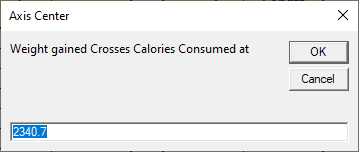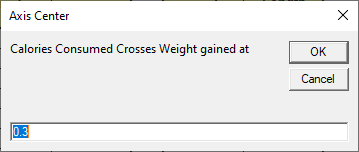A Quadrant Scatter Chart will then be created:## Or Convert a Scatter Plot to a Four-Quadrant Graph Manually

### Scatter Diagram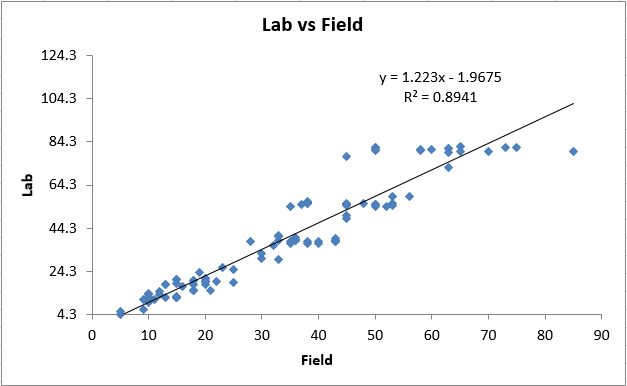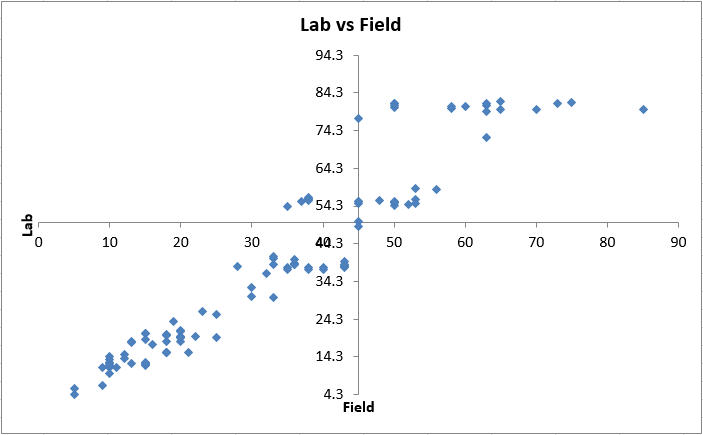### Step by Step Example to Change a Scatter Plot to a Quadrant Graph

1. First, delete the trend line from your scatter diagram.Right click on the X-axis, select Format Axis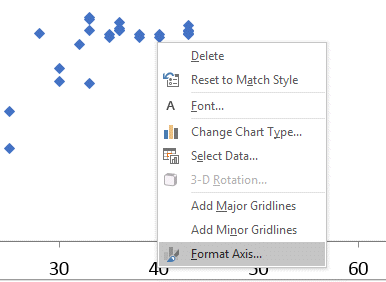Now change the value where you want the vertical Y-axis to cross the X-axis. In this example, we chose 45.Right click on the Y-axis, select Format AxisNow change the value where you want the horizontal X-axis to cross the Y-axis. In this example, we chose 50.4. Once you have updated both the X and Y Axis Values, you should have a XY Scatter Diagram with Four Quadrants!